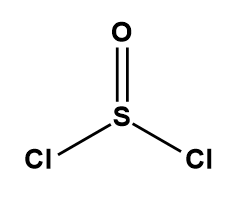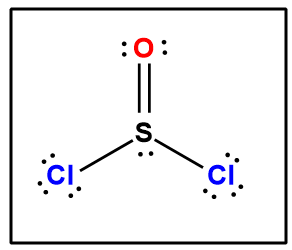# Problem: 1. Write a Lewis structure for each of the following compounds, then answer the following questions about the Lewis structure.Compounds - SOCl2, ethanol (CH3CH2OH), and carbon dioxide.Questions:A.   How many valence electrons does each compound have?B.   How many bonded electrons does each compound have?C.   How many lone pairs of electrons does each compound have?D.   How many single bonds does each compound have?

###### FREE Expert Solution

SOCl2

Step 1: Sulfur (EN = 2.58) is less electronegative than chlorine (EN = 3.16) and oxygen (EN = 3.44) so sulfur is the central atom.

Step 2: The total number of valence electrons present in SOCl2 is:

Group             Valence Electrons

S         6A                   1 × 6 e6 e

O         6A                   1 × 6 e6 e

Cl        7A                   2 × 7 e14 e

Total:  26 valence e

Step 3: The bonding preferences of each element in the molecule is as follows:

Suflur: expanded octet
• Oxygen: prefers to form 2 bonds

• Chlorine: prefers to form 1 bond (when not the central atom)

Let’s first connect each atom while following their bonding preferences:A bond corresponds to 2 electrons shared between two atoms so we currently have 4 × 2 e = 8 val. e, which means we’re still lacking 18 e. We can start adding the missing electrons to the terminal atoms while following the octet rule

The complete Lewis structure is:###### Problem Details

1. Write a Lewis structure for each of the following compounds, then answer the following questions about the Lewis structure.

Compounds - SOCl2, ethanol (CH3CH2OH), and carbon dioxide.

Questions:

A.   How many valence electrons does each compound have?

B.   How many bonded electrons does each compound have?

C.   How many lone pairs of electrons does each compound have?

D.   How many single bonds does each compound have?